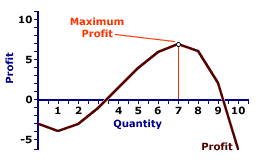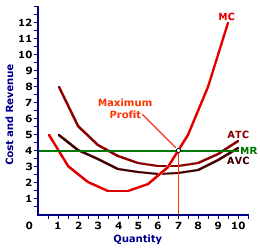Thursday  March 30, 2023
 AmosWEB means Economics with a Touch of Whimsy!WANTS AND NEEDS: These are the unfulfilled desires that motivate human behavior and that when satisfied improve human well-being. They include both physiological or biological requirements for maintaining life (needs) and the psychological desires which make life more enjoyable (wants). However, when push comes to shove, and the nitty gets down to the gritty, it matters very little to markets if people need goods or want goods, so long as they are motivated to buy the goods to satisfy wants and needs.PERFECT COMPETITION, PROFIT MAXIMIZATION:

A perfectly competitive firm is presumed to produce the quantity of output that maximizes economic profit--the difference between total revenue and total cost. This production decision can be analyzed directly with economic profit, by identifying the greatest difference between total revenue and total cost, or by the equality between marginal revenue and marginal cost.
Profit Maximization
Profit CurveTotal CurvesMarginal CurvesThe profit-maximizing level of output is a production level that achieves the greatest level of economic profit given existing market conditions and production cost. For a perfectly competitive firm, this entails adjusting the production level in response to the going market price.

### Three Views

Profit-maximizing output can be identified in one of three ways--directly with economic profit, with a comparison of total revenue and total cost, and with a comparison of marginal revenue and marginal cost.

This exhibit illustrates how it can be identified for a perfectly competitive firm, such as that operated by Phil the zucchini growing gardener. Phil sells zucchinis in a market with gadzillions of other zucchini growers and thus faces a going market price of \$4 for each pound of zucchinis sold.

The top panel presents the profit curve. The middle panel presents total revenue and total cost curves. The bottom panel presents marginal revenue and marginal cost curves. In all three panels, Phil maximizes when producing 7 pounds of zucchinis.

• Profit: First, profit maximization can be illustrated with a direct evaluation of profit. If the profit curve is at its peak, then profit is maximized. In the top panel, the profit curve achieves its highest level at 7 pounds of zucchinis. At other output levels, profit is less.

• Total Revenue and Total Cost: Second, profit maximization can be identified by a comparison of total revenue and total cost. The quantity of output that achieves the greatest difference of total revenue over total cost is profit maximization. In the middle panel, the vertical gap between the total revenue and total cost curves is the greatest at 7 pounds of zucchinis. For smaller or larger output levels, the gap is either less or the total cost curve lies above the total revenue curve.

• Marginal Revenue and Marginal Cost: Third, profit maximization can be identified by a comparison of marginal revenue and marginal cost. If marginal revenue is equal to marginal cost, then profit cannot be increased by changing the level of production. Increasing production adds more to cost than revenue, meaning profit declines. Decreasing production subtracts more from revenue than from cost, meaning profit also declines. In the bottom panel, the marginal revenue and marginal cost curves intersect at 7 pounds of zucchinis. At larger or smaller output levels, marginal cost exceeds marginal revenue or marginal revenue exceeds marginal cost.

### More on the Marginal View

Further analysis of the marginal approach to analyzing profit maximization provides further insight into the short-run production decision of a perfectly competitive firm.

First, consider the logic behind using marginals to identify profit maximization.

1. Marginal revenue indicates how much total revenue changes by producing one more or one less unit of output.

2. Marginal cost indicates how much total cost changes by producing one more or one less unit of output.

3. Profit increases if marginal revenue is greater than marginal cost and profit decreases if marginal revenue is less than marginal cost.

4. Profit neither increases nor decreases if marginal revenue is equal to marginal cost.

5. As such, the production level that equates marginal revenue and marginal cost is profit maximization.
Profit Maximization,
The Marginal ViewWith this in mind, now consider this exhibit to the right, which will eventually contain the marginal revenue and marginal cost curves for Phil's zucchini production.
• Marginal Revenue: Because Phil is a price taker, his marginal revenue curve is a horizontal line. Click the [Marginal Revenue] button to reveal this curve. It is perfectly elastic at the going market price of \$4 per pound of zucchinis.

• Marginal Cost: The marginal cost curve is U-shaped, reflecting the principles of short-run production. Click the [Marginal Cost] button to add this curve to the diagram. It has a negative slope for small amounts of output, then the slope is positive for larger quantities due to the law of diminishing marginal returns.

• Profit Maximization: Profit is maximized at the quantity of output found at the intersection of the marginal revenue and marginal cost curves, which is 7 pounds of zucchinis. Click the [Profit Max] button to highlight this production level. This is the same profit-maximizing level identified using the total revenue and total cost curves and the profit curve.

Consider what results if marginal revenue is not equal to marginal cost:

• If marginal revenue is greater than marginal cost, as is the case for small quantities of output, then the firm can increase profit by increasing production. Extra production adds more to revenue than to cost, so profit increases.

• If marginal revenue is less than marginal cost, as is the case for large quantities of output, then the firm can increase profit by decreasing production. Reducing production reduces revenue less than to it reduces cost, so profit increases.

• If marginal revenue is equal to marginal cost, then the firm cannot increase profit by producing more or less output. Profit is maximized.

 <= PERFECT COMPETITION, PROFIT ANALYSIS PERFECT COMPETITION, REALISM =>Recommended Citation:

PERFECT COMPETITION, PROFIT MAXIMIZATION, AmosWEB Encyclonomic WEB*pedia, http://www.AmosWEB.com, AmosWEB LLC, 2000-2023. [Accessed: March 30, 2023].

Check Out These Related Terms...

Or For A Little Background...

And For Further Study...
Search Again?BLACK DISMALAPOD[What's This?] Today, you are likely to spend a great deal of time driving to a factory outlet wanting to buy either a coffee cup commemorating the first day of spring or a printer that works with your stockpile of ink cartridges. Be on the lookout for telephone calls from long-lost relatives.Your Complete ScopeNatural gas has no odor. The smell is added artificially so that leaks can be detected."The mediocre teacher tells. The good teacher explains. The superior teacher demonstrates. The great teacher inspires."-- William Ward ‚ Texas Wesleyan University AdministratorICCInternational Chamber of CommerceA PEDestrian's Guide Xtra CreditTell us what you think about AmosWEB. Like what you see? Have suggestions for improvements? Let us know. Click the User Feedback link.| | | | | | | | | | |
| | | |

Thanks for visiting AmosWEB